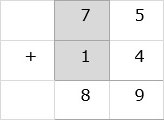# Addition of Two 2-digit Numbers Without Carry

#### Excel add-in with c# VSTO and web

7 Lectures 1.5 hours

#### Word add-in using c# vsto and web types

8 Lectures 1 hours

#### The Ultimate Guide To Add Social Media Login to PHP Web Apps

17 Lectures 2 hours

Add the numbers 36 + 51

### Solution

Step 1:

Line up the numbers vertically.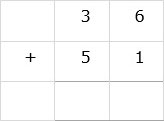Step 2:

We first add the digits in ones column. 6 + 1= 7. 7 is written below ones column.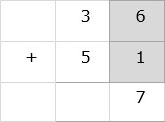Step 3:

Next we add the digits in tens column. 3 + 5 = 8. 8 is written below tens column. So the sum of numbers is 87.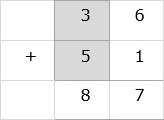Find the sum 75 + 14

### Solution

Step 1:

We line up the numbers vertically.Step 2:

We add the digits in ones column. 5 + 4 = 9. 9 is written below the one column.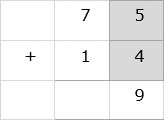Step 3:

Next we add the digits in tens column. 7 + 1 = 8. 8 is written below the tens column. The final sum is 89.